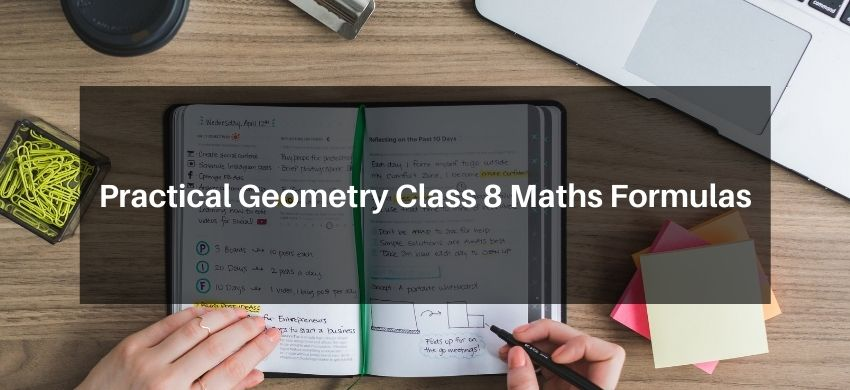Download the eSaral app and start learning from Kota's top IITians and doctors.

# Practical Geometry Class 8 Maths FormulasHey, students are you looking for Practical Geometry Class 8 Maths Formulas? If yes. Then you are at the right place. In this post, I have listed all the formulas of Geometry class 8 that you can use to learn and understand the concepts easily.

If you want to improve your class 8 Math, Geometry concepts, then it is super important for you to learn and understand all the formulas.

By using these formulas you will learn about the Practical Geometry.

With the help of these formulas, you can revise the entire chapter easily.

## Practical Geometry Class 8 Maths Formulas

• Square $a^{2}$
• Rectangle $a \times b$
• Circle $\pi r^{2}$
• Ellipse $\pi r_{1} r_{2}$
• Triangle $1 / 2 \times b \times h$